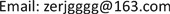1. 引言

2. 预备知识

‖ A ‖ ∗ : = 1 n 3 ∑ i = 1 n 3 ‖ A ¯ ( i ) ‖ ∗ (1)

‖ X ‖ r = ‖ X ‖ ∗ − max A ∗ A T = I B ∗ B T = I t r ( A ∗ X ∗ B ) (2)

max i = 1 , ⋯ , n 1 ‖ U ∗ ∗ e i ∘ ‖ F ≤ μ r n 1 n 3 (3)

max j = 1 , ⋯ , n 2 ‖ V ∗ ∗ e j ∘ ‖ F ≤ μ r n 2 n 3 (4)

‖ U ∗ V ∗ ‖ ∞ ≤ μ r n 1 n 2 n 2 3 (5)

A = U ∗ S ∗ V ∗ (6)

U ∈ ℝ n 1 × n 1 × n 3 , V ∈ ℝ n 2 × n 2 × n 3 是正交的， S ∈ ℝ n 1 × n 2 × n 3 是f-对角张量。

3. 截断核范数的鲁棒主成分分析3.1. 模型的建立

3.2. 模型的求解

min X     ‖ X ‖ ∗ − T r ( A l W B l T ) + λ ‖ E ‖ 1     s .t .     L = W ,   X = L + E (10)

L ( L , E , W , Y 1 , Y 2 ) = ‖ L ‖ ∗ − T r ( A l W B l T ) + λ ‖ E ‖ 1 + 〈 Y 1 , L + E − X 〉     + 〈 Y 2 , L − W 〉 + μ 2 ( ‖ L + E − X ‖ F 2 + ‖ L − W ‖ F 2 ) (11)

L k + 1 = arg min L   ‖ L ‖ ∗ + 〈 Y 1 , k , L + E k − X 〉 + 〈 Y 2 , k , L − W k 〉 + μ 2 ( ‖ L + E k − X ‖ F 2 + ‖ L − W k ‖ F 2 ) = arg min L   ‖ L ‖ ∗ + μ ‖ L + 1 2 ( E k − X − W k + 1 μ ( Y 1 k + Y 2 k ) ) ‖ F 2 (12)

E k + 1 = arg min E λ ‖ E ‖ 1 + 〈 Y 1 k , L k + 1 + E − X 〉 + μ 2 ‖ L k + 1 + E − X ‖ F 2 = arg min E λ ‖ E ‖ 1 + μ 2 ‖ E + ( L k + 1 − X + 1 μ Y 1 , k ) ‖ F 2 (14)

E k + 1 = S λ μ k ( X − L k + 1 − 1 μ Y 1 , k ) (15)

W k + 1 = arg min W     − T r ( A l W B l T ) + 〈 Y 2 k , L k + 1 − W 〉 + μ 2 ‖ L k + 1 − W ‖ F 2 (16)

(16)式为关于 W 的二次项，令其导数为0，可得到闭式解。去掉无关项得：

W k + 1 = arg min W     − T r ( A l W B l T ) + μ 2 ‖ W ‖ F 2 + 〈 − Y 2 k , W 〉 + μ 〈 − L k + 1 , W 〉 (17)

− A l B l T + μ W − Y 2 k − μ L k + 1 = 0 (18)

W = L k + 1 + 1 μ ( A l B l T + Y 2 k ) (19)

Y 1 k + 1 = Y 1 k + μ ( L k + 1 + E k + 1 − X ) Y 2 k + 1 = Y 2 k + μ ( L k + 1 − W k + 1 ) (20)

4. 实验

Data denoised by each algorith

TRPCA22.09364.424630.16
TNNR23.60170.424320.14
SNN26.4086.512130.12
TNNRPCA30.8743.131420.11

5. 结论